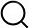# Also in the Article

Statistical analysis
This protocol is extracted from research article:
The deubiquitinase UCHL1 regulates cardiac hypertrophy by stabilizing epidermal growth factor receptor

Procedure

Statistical calculations were performed using SPSS version 16.0 (SPSS Inc., Chicago, IL, USA). Sample numbers are shown in the figures and figure legends and in Materials and Methods. First, the normality test was performed. If each group satisfied the normality and the variance between the groups was equal, we used Student’s t test to determine the significant difference between two groups, and one-way analysis of variance (ANOVA) with the Bonferroni post hoc test for comparisons among more than two groups; if the above conditions were not met, we used the nonparametric Mann-Whitney U test. Statistical differences in means were considered statistically significant at P < 0.05.

Note: The content above has been extracted from a research article, so it may not display correctly.

Q&A